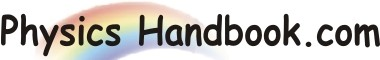HOME TOPICS DEFINITIONS TABLES LAWS INVENTIONS EXPERIMENTS QUIZ VIDEOS
 A B C D E F G H I J K L M N O P Q R S T U V W X Y Z
Young's Modulus
Young's modulus is number representing the ratio of stress to strain for a wire or bar of a given substance.
Young's modulus is usually measured in pounds per square inch or dynes per square centimeter.
According to Hooke's law the strain is proportional to stress, and therefore the ratio of the two is a constant that is commonly used to indicate the elasticity of the substance.
It is also known as the Young modulus, modulus of elasticity, elastic modulus or tensile modulus.
Young's modulus is named after Thomas Young, the 18th century British scientist.
Young's modulus is the ratio of stress, which has units of pressure, to strain, which is dimensionless; therefore Young's modulus itself has units of pressure.
Young's modulus is represented by the slope E = ΔS / Δε of the initial straight segment of the stress-strain diagram. More correctly, E is a measure of stiffness, having the same units as stress: pounds per square inch or pascals.
When stress and strain are not directly proportional, E may be represented as the slope of the tangent or the slope of the secant connecting two points on the stress-strain curve.